# Maharashtra Board Class 10 Maths Solutions Chapter 2 Quadratic Equations Practice Set 2.3

## Maharashtra State Board Class 10 Maths Solutions Chapter 2 Quadratic Equations Practice Set 2.3

Question 1.
Solve the following quadratic equations by completing the square method.
1. x2 + x – 20 = 0
2. x2 + 2x – 5 = 0
3. m2 – 5m = -3
4. 9y2 – 12y + 2 = 0
5. 2y2 + 9y + 10 = 0
6. 5x2 = 4x + 7
Solution:
1. x2 + x – 20 = 0
If x2 + x + k = (x + a)2, then
x2 + x + k = x2 + 2ax + a2
Comparing the coefficients, we get
1 = 2a and k = a2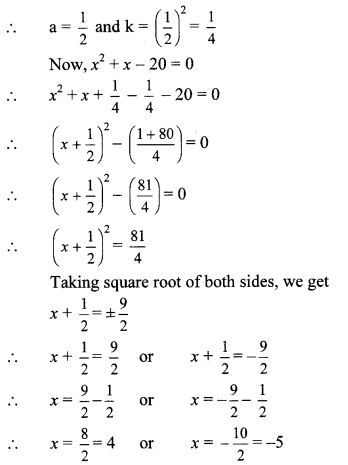∴ The roots of the given quadratic equation are 4 and -5.

2. x2 + 2x – 5 = 0
If x2 + 2x + k = (x + a)2, then
x2 + 2x + k = x2 + 2ax + a2
Comparing the coefficients, we get
2 = 2a and k = a2
∴ a = 1 and k = (1)2 = 1
Now, x2 + 2x – 5 = 0
∴ x2 + 2x + 1 – 1 – 5 = 0
∴ (x + 1)2 – 6 = 0
∴ (x + 1)2 = 6
Taking square root of both sides, we get
x + 1 = ± √6
∴ x + 1 √6 or x + 1 = √6
∴ x = √6 – 1 or x = -√6 – 1
∴ The roots of the given quadratic equation are √6 -1 and – √6 -1.

3. m2 – 5m = -3
∴ m2 – 5m + 3 = 0
If m2 – 5m + k = (m + a)2, then
m2 – 5m + k = m2 + 2am + a2
Comparing the coefficients, we get
-5 = 2a and k = a2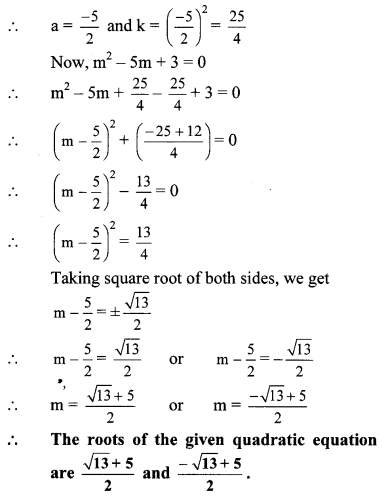4. 9y2 – 12y + 2 = 0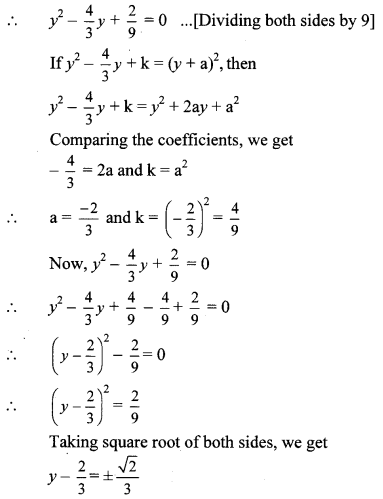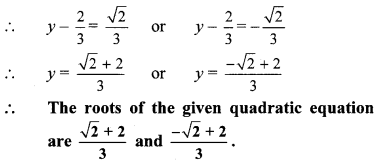5. 2y2 + 9y + 10 = 0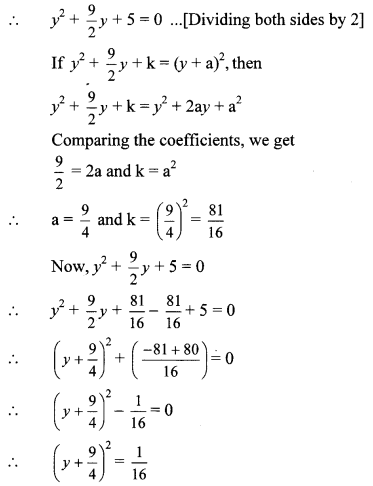Taking square root of both sides, we get∴ The roots of the given quadratic equation are -2 and $$\frac { -5 }{ 2 }$$.

6. 5x2 = 4x + 7
∴ 5x2 – 4x – 7 = 0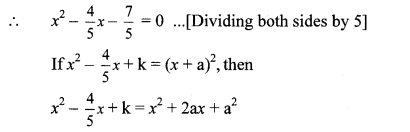Comparing the coefficients, we get